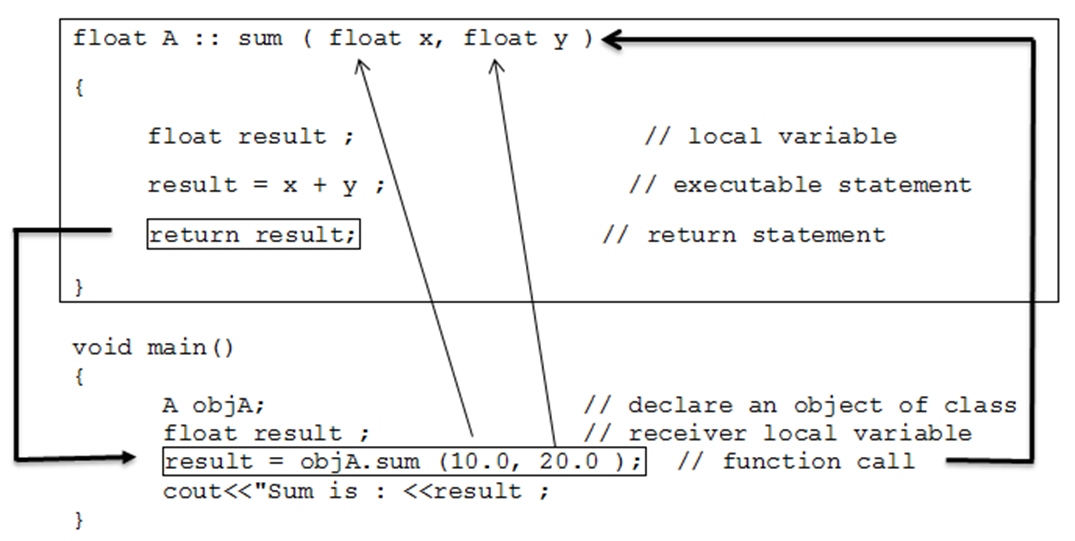• Function in C++ program is similar to function in C program.
• Function provides the top-down structured programming approach.
• It also provides re-usability that means we can use the same function multiple times. Functions in C++ contains mainly:
1. Function Declaration
2. Function Definition
3. Function Call
• When a function is called, control transfers to the function definition section then body of the function is executed statement by statement.
• After completing the execution of called function control transfers back to the calling function.
• C++ adds some new features in function such as function overloading that means we can define multiple functions with same name. Read more about Function overloading later. Functions in C++ also provides the facility of default argument that mean if no argument passed, your function will accept the default value.

### Function Declaration (Function Prototyping):

• The function prototype describes the function interface to the compiler by providing details about the total no. of arguments, types of arguments, and return value. Function declaration is placed under the public section in class declaration.
• Function prototyping uses the template during declaring and defining function. When function is called compiler uses template to check correct number of argument, types of argument, and return type. In function prototyping during declaration the no. of arguments, types of arguments, and return value is specified. We can declare function using the following syntax:

Syntax:

``return-type  function-name (data-type argument1, data-type argument2...);``
• Function prototype has the following three parts:
• Return type : it specifies return type of the function that means type of the data that a function will return such as int, float, char etc.
• Function that does not return any value will have return type as void.
• Function Name: it is the name of the function and it should be any meaningful name.
• It should follow all naming convention rules.
• Some examples of function names are display, show, calculate etc.
• Argument List: argument list specifies list of all arguments required for the function to perform the desired task.
• Arguments are separated by comma. It may include both data type and argument name but normally only data type is provided.
• The following is an example of function prototype for doing sum of two float values:
``float  sum (float, float );  OR float sum (float x, float y) ;``
• Where, int is a return type, sum is the name of the function and int x, int y are the argument list.
• The argument in function definition is referred to as the formal argument while the argument provided during the function call is known as the actual argument.
• C++ also allows the empty argument-list in function declaration.
``````void     display( );
void     display(void);   // Both are same``````
• In C++ programming empty argument-list means we are not passing any argument. To pass any number of arguments we are using ellipses in the function declaration:
``void show (...) ;``

### Function Definition:

• Function Definition can be placed either inside the class definition under the public section or outside the class definition. If it is placed inside the class definition, we need not to declare function instead function definition is placed directly as shown below.
``````public :
data-type variable-name;
.
.
.

// Function definition inside class
return-value   function-name (data-type argument1, data-type argument2, …)
{
// function code;
}``````
• Normally, function definition is included inside the class definition for inline functions.  If it is written outside the class definition, then we have to use function prototype inside the class definition and function definition is placed outside the class definition with scope resolution operator (::) using the following syntax:
``````// Function definition outside class with scope resolution operator
return-value   class-name ::  function-name (data-type argument1, data-type argument2, …)
{
//      function code;
}``````

### Function Call:

• Once we are done with function declaration and function definition, we can use that function by calling it on object.
• A function can be called simply by using name of the function followed by a list of Actual parameters (Arguments) if any.
• The following example calls sum() function that we defined earlier.
``````void main()
{
A objA;                          // declare an object of class
float result ;                   // receiver local variable
result = objA.sum (10.0, 20.0 );     // function call
cout<<"Sum is : <<result ;
}``````
• When the compiler encounters a function call, the control is transferred to the function definition and executes the function line by line and a computed value is returned with the help of return statement. The returned value is assigned to the receiver variable. This is illustrated as below:• Arguments that passed in function call and arguments receiving in function definition should have the same data type.
• For example in above function call sum (10.0, 20.0) we have passed arguments of float type and inside function definition those float type arguments are received by float type variable x and y respectively. In short, type of arguments in function call and function definition should be same.
• The complete program with function implementation will look as below:
``````#include “iostream.h”
#include “conio.h”

class A
{
private :
float x, y;
public :
float sum (float, float);
void display();
};

float A :: sum ( float x, float y )
{
float result ;                   // local variable
result = x + y ;                // executable statement
return result ;                // return statement
}
void main()
{
A objA;                     // declare an object of class
float result ;              // receiver local variable
result = objA.sum (10.0, 20.0 );  // function call
cout<<"Sum is : <<result ;
}``````